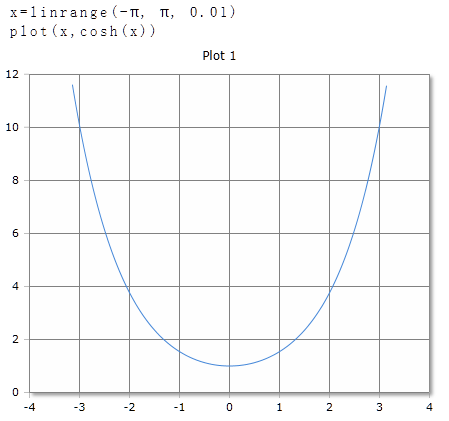# Cosh Function

Computes the hyperbolic cosine

## Description

The function $$Cosh$$ berechnet den hyperbolischen Kosinus des Arguments.

$$Cosh$$ returns the hyperbolic cosine of real or complex numbers. The argument can be a single number or a data field. For data fields, the hyperbolic cosine of each element is calculated and the results are returned in a data field of equal size.

## Cosh for real numbers

### Parameter

The angle is given in degrees (full circle = 360°) or radians / radians (full circle = 2 · p). The unit of measure used is set in the toolbar with the DEG or RAD buttons or with an optional parameter. The setting in the toolbar applies to the entire worksheet.

### Optional Parameter

Optionally, a second parameter can be specified with the keywords DEG or RAD to set the unit of measure for this function call. The specification of the parameter has priority over the global setting in the toolbar. It allows you to use different units of measure on a spreadsheet, regardless of the default toolbar setting.

### Syntax

Cosh (value)

Cosh (value, DEG)

### Example## Cosh for complex numbers

For complex numbers, the result is always given as radians, regardless of the default setting in the toolbar. The result is also a complex number.

Cosh (re + im)

### Example

Cosh(3 + 4i)= -6.58-7.58i